GeeksforGeeks App
Open AppBrowser
Continue

R language is mostly used for statistics and data analytics purposes to represent the data graphically in the software. To represent those data graphically, charts and graphs are used in R.

## R – graphs

There are hundreds of charts and graphs present in R. For example, bar plot, box plot, mosaic plot, dot chart, coplot, histogram, pie chart, scatter graph, etc.

### Types of R – Charts

• Bar Plot or Bar Chart
• Pie Diagram or Pie Chart
• Histogram
• Scatter Plot
• Box Plot

### Bar Plot or Bar Chart

Bar plot or Bar Chart in R is used to represent the values in data vector as height of the bars. The data vector passed to the function is represented over y-axis of the graph. Bar chart can behave like histogram by using table() function instead of data vector.

Syntax: barplot(data, xlab, ylab)

where:

• data is the data vector to be represented on y-axis
• xlab is the label given to x-axis
• ylab is the label given to y-axis

Note: To know about more optional parameters in barplot() function, use the below command in R console:

`help("barplot")`

Example:

## R

 `# defining vector``x <- ``c``(7, 15, 23, 12, 44, 56, 32)` `# output to be present as PNG file``png``(file = ``"barplot.png"``)` `# plotting vector``barplot``(x, xlab = ``"GeeksforGeeks Audience"``,``        ``ylab = ``"Count"``, col = ``"white"``,``        ``col.axis = ``"darkgreen"``,``        ``col.lab = ``"darkgreen"``)` `# saving the file``dev.off``()`

Output: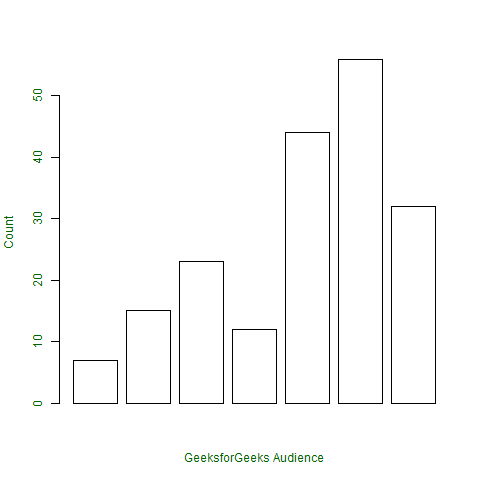### Pie Diagram or Pie Chart

Pie chart is a circular chart divided into different segments according to the ratio of data provided. The total value of the pie is 100 and the segments tell the fraction of the whole pie. It is another method to represent statistical data in graphical form and pie() function is used to perform the same.

Syntax: pie(x, labels, col, main, radius)

where,

• x is data vector
• labels shows names given to slices
• col fills the color in the slices as given parameter
• main shows title name of the pie chart
• radius indicates radius of the pie chart. It can be between -1 to +1

Note: To know about more optional parameters in pie() function, use the below command in the R console:

`help("pie")`

Example:

Assume, vector x indicates the number of articles present on the GeeksforGeeks portal in categories names(x)

## R

 `# defining vector x with number of articles``x <- ``c``(210, 450, 250, 100, 50, 90)` `# defining labels for each value in x``names``(x) <- ``c``(``"Algo"``, ``"DS"``, ``"Java"``, ``"C"``, ``"C++"``, ``"Python"``)` `# output to be present as PNG file``png``(file = ``"piechart.png"``)` `# creating pie chart``pie``(x, labels = ``names``(x), col = ``"white"``,``main = ``"Articles on GeeksforGeeks"``, radius = -1,``col.main = ``"darkgreen"``)` `# saving the file``dev.off``()`

Output: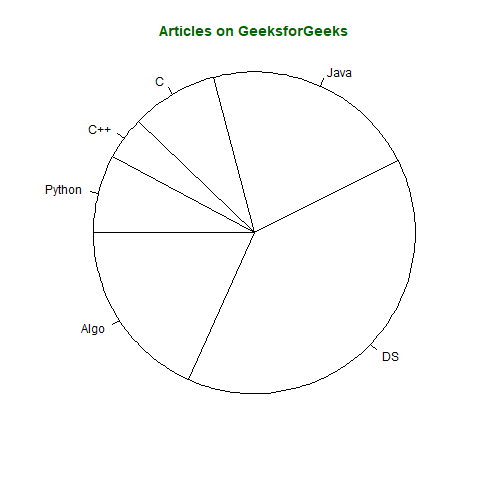Pie chart in 3D can also be created in R by using following syntax but requires plotrix library.

Note: To know about more optional parameters in pie3D() function, use below command in R console:

`help("pie3D")`

Example:

## R

 `# importing library plotrix for pie3D()``library``(plotrix)` `# defining vector x with number of articles``x <- ``c``(210, 450, 250, 100, 50, 90)` `# defining labels for each value in x``names``(x) <- ``c``(``"Algo"``, ``"DS"``, ``"Java"``, ``"C"``, ``"C++"``, ``"Python"``)` `# output to be present as PNG file``png``(file = ``"piechart3d.png"``)` `# creating pie chart``pie3D``(x, labels = ``names``(x), col = ``"white"``,``main = ``"Articles on GeeksforGeeks"``,``labelcol = ``"darkgreen"``, col.main = ``"darkgreen"``)` `# saving the file``dev.off``()`

Output: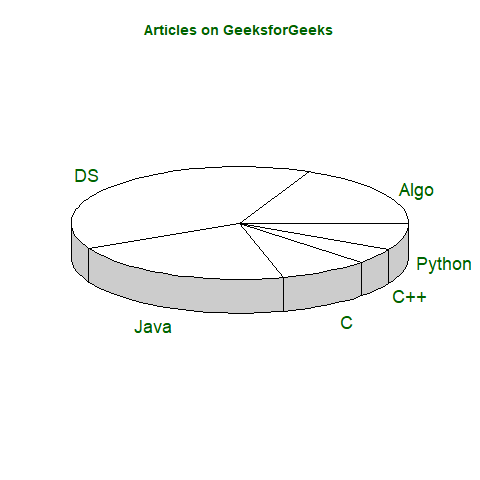### Histogram

Histogram is a graphical representation used to create a graph with bars representing the frequency of grouped data in vector. Histogram is same as bar chart but only difference between them is histogram represents frequency of grouped data rather than data itself.

Syntax: hist(x, col, border, main, xlab, ylab)

where:

• x is data vector
• col specifies the color of the bars to be filled
• border specifies the color of border of bars
• main specifies the title name of histogram
• xlab specifies the x-axis label
• ylab specifies the y-axis label

Note: To know about more optional parameters in hist() function, use below command in R console:

`help("hist")`

Example:

## R

 `# defining vector``x <- ``c``(21, 23, 56, 90, 20, 7, 94, 12,``    ``57, 76, 69, 45, 34, 32, 49, 55, 57)` `# output to be present as PNG file``png``(file = ``"hist.png"``)` `# hist(x, main = "Histogram of Vector x",``        ``xlab = ``"Values"``,``        ``col.lab = ``"darkgreen"``,``        ``col.main = ``"darkgreen"``)` `# saving the file``dev.off``()`

Output: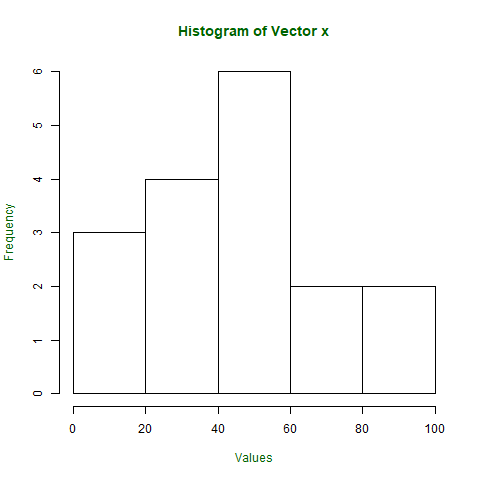### Scatter Plot

A Scatter plot is another type of graphical representation used to plot the points to show relationship between two data vectors. One of the data vectors is represented on x-axis and another on y-axis.

Syntax: plot(x, y, type, xlab, ylab, main)

Where,

• x is the data vector represented on x-axis
• y is the data vector represented on y-axis
• type specifies the type of plot to be drawn. For example, “l” for lines, “p” for points, “s” for stair steps, etc.
• xlab specifies the label for x-axis
• ylab specifies the label for y-axis
• main specifies the title name of the graph

Note: To know about more optional parameters in plot() function, use the below command in R console:

`help("plot")`

Example:

## R

 `# taking input from dataset Orange already``# present in R``orange <- Orange[, ``c``(``'age'``, ``'circumference'``)]` `# output to be present as PNG file``png``(file = ``"plot.png"``)` `# plotting``plot``(x = orange\$age, y = orange\$circumference, xlab = ``"Age"``,``ylab = ``"Circumference"``, main = ``"Age VS Circumference"``,``col.lab = ``"darkgreen"``, col.main = ``"darkgreen"``,``col.axis = ``"darkgreen"``)` `# saving the file``dev.off``()`

Output: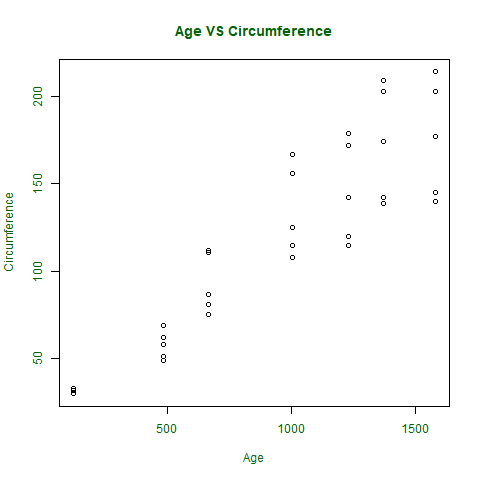If a scatter plot has to be drawn to show the relation between 2 or more vectors or to plot the scatter plot matrix between the vectors, then pairs() function is used to satisfy the criteria.

Syntax: pairs(~formula, data)

where,

• ~formula is the mathematical formula such as ~a+b+c
• data is the dataset form where data is taken in formula

Note: To know about more optional parameters in pairs() function, use the below command in R console:

`help("pairs")`

Example :

## R

 `# output to be present as PNG file``png``(file = ``"plotmatrix.png"``)` `# plotting scatterplot matrix``# using dataset Orange``pairs``(~age + circumference, data = Orange,``col.axis = ``"darkgreen"``)` `# saving the file``dev.off``()`

Output: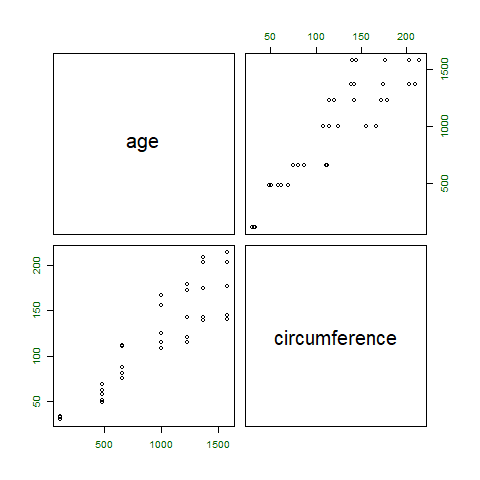### Box Plot

Box plot shows how the data is distributed in the data vector. It represents five values in the graph i.e., minimum, first quartile, second quartile(median), third quartile, the maximum value of the data vector.

Syntax: boxplot(x, xlab, ylab, notch)

where,

• x specifies the data vector
• xlab specifies the label for x-axis
• ylab specifies the label for y-axis
• notch, if TRUE then creates notch on both the sides of the box

Note: To know about more optional parameters in boxplot() function, use the below command in R console:

`help("boxplot")`

Example:

## R

 `# defining vector with ages of employees``x <- ``c``(42, 21, 22, 24, 25, 30, 29, 22,``    ``23, 23, 24, 28, 32, 45, 39, 40)` `# output to be present as PNG file``png``(file = ``"boxplot.png"``)` `# plotting``boxplot``(x, xlab = ``"Box Plot"``, ylab = ``"Age"``,``col.axis = ``"darkgreen"``, col.lab = ``"darkgreen"``)` `# saving the file``dev.off``()`

Output: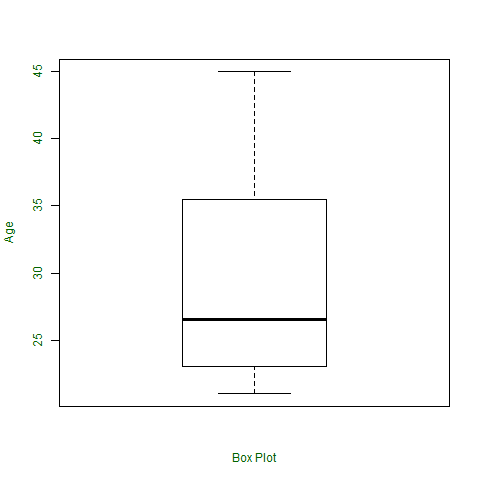My Personal Notes arrow_drop_up